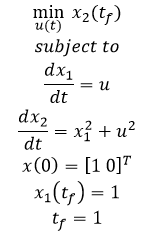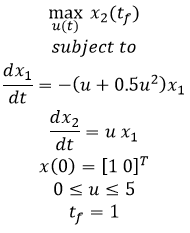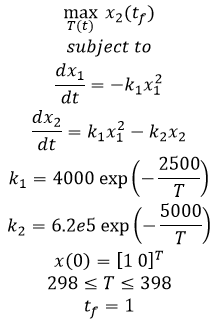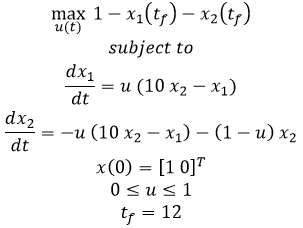## Main.DynamicControl History

November 17, 2021, at 12:48 AM by 10.35.117.248 -
Changed line 111 from:

plt.plot(m.time,p.value,'b-',LineWidth=2)

to:

plt.plot(m.time,p.value,'b-',lw=2)

Changed line 114 from:

plt.plot(m.time,v.value,'r--',LineWidth=2)

to:

plt.plot(m.time,v.value,'r--',lw=2)

(:toggle hide gekko button show="Show GEKKO (Python) Code":) (:div id=gekko:) (:source lang=python:)

1. Import packages

import numpy as np from random import random from gekko import GEKKO import matplotlib.pyplot as plt

1. Build model
2. initialize GEKKO model

m = GEKKO()

1. time

m.time = np.linspace(0,20,41)

1. constants

mass = 500

1. Parameters

b = m.Param(value=50) K = m.Param(value=0.8)

1. Manipulated variable

p = m.MV(value=0, lb=0, ub=100)

1. Controlled Variable

v = m.CV(value=0)

1. Equations

m.Equation(mass*v.dt() == -v*b + K*b*p)

1. Tuning
2. global

m.options.IMODE = 6 #control

1. MV tuning

p.STATUS = 1 #allow optimizer to change p.DCOST = 0.1 #smooth out gas pedal movement p.DMAX = 20 #slow down change of gas pedal

1. CV tuning
2. setpoint

v.STATUS = 1 #add the SP to the objective m.options.CV_TYPE = 2 #L2 norm v.SP = 40 #set point v.TR_INIT = 1 #setpoint trajectory v.TAU = 5 #time constant of setpoint trajectory

1. Solve

m.solve()

1. Plot solution

plt.figure() plt.subplot(2,1,1) plt.plot(m.time,p.value,'b-',LineWidth=2) plt.ylabel('gas') plt.subplot(2,1,2) plt.plot(m.time,v.value,'r--',LineWidth=2) plt.ylabel('velocity') plt.xlabel('time') plt.show() (:sourceend:) (:divend:)

Changed line 17 from:

Objective: Implement a model predictive controller that automatically regulates vehicle velocity. Implement the controller in Excel, MATLAB, Python, or Simulink and tune the controller for acceptable performance. Discuss factors that may be import for evaluating controller performance. Estimated time: 1 hour

to:

Objective: Implement a model predictive controller that automatically regulates vehicle velocity. Implement the controller in Excel, MATLAB, Python, or Simulink and tune the controller for acceptable performance. Discuss factors that may be important for evaluating controller performance. Estimated time: 1 hour

May 18, 2015, at 04:01 PM by 45.56.3.184 -
Changed line 17 from:

Objective: Implement a model predictive controller that automatically regulates vehicle velocity. Implement the controller in MATLAB, Python, and Simulink and tune the controller for acceptable performance. Discuss factors that may be import for evaluating controller performance. Estimated time: 2 hours

to:

Objective: Implement a model predictive controller that automatically regulates vehicle velocity. Implement the controller in Excel, MATLAB, Python, or Simulink and tune the controller for acceptable performance. Discuss factors that may be import for evaluating controller performance. Estimated time: 1 hour

May 18, 2015, at 03:57 PM by 45.56.3.184 -
Deleted lines 11-12:

#### Model Predictive Control Example

May 18, 2015, at 03:55 PM by 45.56.3.184 -
Changed lines 21-22 from:
1. The dynamic relationship between a vehicle gas pedal position (MV) and velocity (CV) is given by the following set of conditions and a single dynamic equation.
to:

The dynamic relationship between a vehicle gas pedal position (MV) and velocity (CV) is given by the following set of conditions and a single dynamic equation.

Changed line 36 from:
1. Discuss the controller performance and how it could be tuned to meet multiple objectives including:
to:

Discuss the controller performance and how it could be tuned to meet multiple objectives including:

May 18, 2015, at 03:55 PM by 45.56.3.184 -
Changed lines 15-16 from:

A method to solve dynamic control problems is by numerically integrating the dynamic model at discrete time intervals, much like measuring a physical system at particular time points. The numerical solution is compared to a desired trajectory and the difference is minimized by adjustable parameters in the model that may change at every time step. The first control action is taken and then the entire process is repeated at the next time instance. The process is repeated because objective targets may change or updated measurements may have adjusted parameter or state estimates. Excel, MATLAB, Python, and Simulink are used in the following example to both solve the differential equations that describe the velocity of a vehicle as well as minimize the control objective function.

to:

A method to solve dynamic control problems is by numerically integrating the dynamic model at discrete time intervals, much like measuring a physical system at particular time points. The numerical solution is compared to a desired trajectory and the difference is minimized by adjustable parameters in the model that may change at every time step. The first control action is taken and then the entire process is repeated at the next time instance. The process is repeated because objective targets may change or updated measurements may have adjusted parameter or state estimates.

#### Exercise

Objective: Implement a model predictive controller that automatically regulates vehicle velocity. Implement the controller in MATLAB, Python, and Simulink and tune the controller for acceptable performance. Discuss factors that may be import for evaluating controller performance. Estimated time: 2 hours

1. The dynamic relationship between a vehicle gas pedal position (MV) and velocity (CV) is given by the following set of conditions and a single dynamic equation.
 Constants
m = 500 ! Mass (kg)
Parameters
b = 50  ! Resistive coefficient (N-s/m)
K = 0.8 ! Gain (m/s-%pedal)
p = 0 >= 0 <= 100  ! Gas pedal position (%)
Variables
v = 0 ! initial condition
Equations
m * \$v = -v * b + K * b * p


Implement a model predictive controller that adjusts gas pedal position to regulate velocity. Start at an initial vehicle velocity of 0 m/s and accelerate to a velocity of 40 m/s.

1. Discuss the controller performance and how it could be tuned to meet multiple objectives including:
• minimize travel time
• remain within speed limits
• improve vehicle fuel efficiency
• discourage excessive gas pedal adjustments
• do not accelerate excessively

There is no need to implement these advanced objectives in simulation for this second part of the exercise, only discuss the possible competing objectives.

#### Solution

Excel, MATLAB, Python, and Simulink are used in this example to both solve the differential equations that describe the velocity of a vehicle as well as minimize the control objective function.

Deleted line 54:
May 18, 2015, at 03:28 PM by 45.56.3.184 -
Deleted lines 22-45:

#### Exercise

Objective: Set up and solve several dynamic optimization benchmark problems1. Create a program to optimize and display the results. Estimated Time (each): 30 minutes

• Example 1a - Nonlinear, unconstrained, minimize final state• Example 1b - Nonlinear, unconstrained, minimize final state with terminal constraint• Example 2 - Nonlinear, constrained, minimize final state• Example 3 - Tubular reactor with parallel reaction• Example 4 - Batch reactor with consecutive reactions A->B->CExample 5 - Catalytic plug flow reactor with A->B->C#### SolutionDynamic Optimization Benchmarks in MATLAB and Python

#### References

1. M. Čižniar, M. Fikar, M.A. Latifi: A MATLAB Package for Dynamic Optimisation of Processes, 7th International Scientific – Technical Conference – Process Control 2006, June 13 – 16, 2006, Kouty nad Desnou, Czech Republic. Article
April 28, 2015, at 05:52 PM by 45.56.3.184 -
Changed lines 25-26 from:

Objective: Set up and solve several dynamic optimization benchmark problems1. Create a program to optimize and display the results. Estimated Time (each): 30 minutes

to:

Objective: Set up and solve several dynamic optimization benchmark problems1. Create a program to optimize and display the results. Estimated Time (each): 30 minutes

Changed line 42 from:Dynamic Optimization Benchmarks in MATLAB and Python Δ
to:Dynamic Optimization Benchmarks in MATLAB and Python
April 28, 2015, at 05:50 PM by 45.56.3.184 -
Changed line 46 from:
1. M. Čižniar, M. Fikar, M.A. Latifi: A MATLAB Package for Dynamic Optimisation of Processes, 7th International Scientific – Technical Conference – Process Control 2006, June 13 – 16, 2006, Kouty nad Desnou, Czech Republic.
to:
1. M. Čižniar, M. Fikar, M.A. Latifi: A MATLAB Package for Dynamic Optimisation of Processes, 7th International Scientific – Technical Conference – Process Control 2006, June 13 – 16, 2006, Kouty nad Desnou, Czech Republic. Article
April 27, 2015, at 11:11 PM by 10.5.113.179 -

#### Exercise

Objective: Set up and solve several dynamic optimization benchmark problems1. Create a program to optimize and display the results. Estimated Time (each): 30 minutes

• Example 1a - Nonlinear, unconstrained, minimize final state• Example 1b - Nonlinear, unconstrained, minimize final state with terminal constraint• Example 2 - Nonlinear, constrained, minimize final state• Example 3 - Tubular reactor with parallel reaction• Example 4 - Batch reactor with consecutive reactions A->B->CExample 5 - Catalytic plug flow reactor with A->B->C#### SolutionDynamic Optimization Benchmarks in MATLAB and Python Δ

#### References

1. M. Čižniar, M. Fikar, M.A. Latifi: A MATLAB Package for Dynamic Optimisation of Processes, 7th International Scientific – Technical Conference – Process Control 2006, June 13 – 16, 2006, Kouty nad Desnou, Czech Republic.
April 04, 2015, at 02:03 PM by 45.56.12.124 -
Changed line 17 from:
to:MPC in Excel, MATLAB, Python, and Simulink
April 03, 2015, at 01:40 AM by 10.24.17.95 -
April 02, 2015, at 11:12 PM by 10.10.145.116 -
Changed line 18 from:

<iframe width="560" height="315" src="https://www.youtube.com/embed/umxAfu44kWo?rel=0" frameborder="0" allowfullscreen></iframe>

to:

<iframe width="560" height="315" src="https://www.youtube.com/embed/dqm2OqXYLR8?rel=0" frameborder="0" allowfullscreen></iframe>

April 02, 2015, at 04:48 PM by 45.56.12.124 -

(:html:) <iframe width="560" height="315" src="https://www.youtube.com/embed/DFqOf5wbQtc?rel=0" frameborder="0" allowfullscreen></iframe> (:htmlend:)

April 02, 2015, at 04:19 PM by 45.56.12.124 -
Changed lines 5-16 from:

Dynamic control is a method to use model predictions to plan an optimized future trajectory for time-varying systems. It is often referred to as Model Predictive Control (MPC) or Dynamic Optimization.

to:

Dynamic control is a method to use model predictions to plan an optimized future trajectory for time-varying systems. It is often referred to as Model Predictive Control (MPC) or Dynamic Optimization.

#### Model Predictive Control Example

A method to solve dynamic control problems is by numerically integrating the dynamic model at discrete time intervals, much like measuring a physical system at particular time points. The numerical solution is compared to a desired trajectory and the difference is minimized by adjustable parameters in the model that may change at every time step. The first control action is taken and then the entire process is repeated at the next time instance. The process is repeated because objective targets may change or updated measurements may have adjusted parameter or state estimates. Excel, MATLAB, Python, and Simulink are used in the following example to both solve the differential equations that describe the velocity of a vehicle as well as minimize the control objective function.

(:html:) <iframe width="560" height="315" src="https://www.youtube.com/embed/umxAfu44kWo?rel=0" frameborder="0" allowfullscreen></iframe> (:htmlend:)